# I have a question that if i have a graph that is bipartite but not a perfect matching how do i justify that its not a pe...

I have a question that if i have a graph that is bipartite but not a perfect matching how do i justify that its not a perfect matching by using halls theorem? Whats the explanation?

Imagine that you have 4 students looking for a job, and 4 positions available to fill. Not all students are equal — some are smarter than others. So the companies want to hire only the smartest students.

(Students are happy with any job they can get)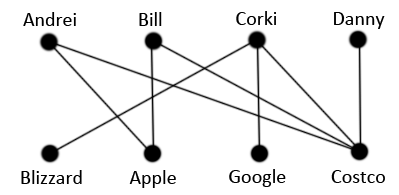In this diagram, a bipartite graph, the students are at the top and the companies are at the bottom. A student and a company is connected if the company wants to hire the student. For example, Costco will hire any student, so Costco is connected to Andrei, Bill, Corki, and Danny.

Hall’s Theorem tells us when we can have the perfect matching:

Supposeis a bipartite graph with bipartition. There is a matching that coversif and only if for every subset,whereis the number of neighbors of.

If you look closely at the diagram, you’ll notice that it doesn’t quite work: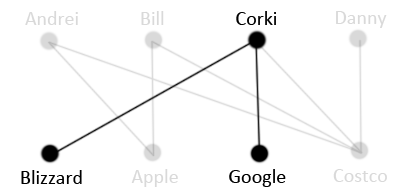Both Blizzard and Google want to hire Corki and only Corki. But Corki can only work for one company! So the whole thing collapses; the matching fails.

Let’s rewrite Hall’s condition in the context of students and jobs:

For a set ofcompanies, denoteto mean the number of students that at least one of these companies want. Iffor every set of companies, then a matching is possible. Otherwise, the matching fails.

Here, a set of {Blizzard, Google} consists of 2 companies, but only one student, Corki, is wanted by either company. Since 1 < 2, the matching fails.

A perfect matching is a matching in which each node has exactly one edge incident on it.

In the above example we have a graph that is bipartite but not a perfect matching.We have proved it using halls therom.

##### Add Answer of: I have a question that if i have a graph that is bipartite but not a perfect matching how do i justify that its not a pe...
Similar Homework Help Questions
• ### (i) Define the term bipartite graph. How can a bipartite graph be characterized in terms of its c...

(i) Define the term bipartite graph. How can a bipartite graph be characterized in terms of its cycles (ii) What does the Matching Theorem say about certain bipartite graphs? (iii) Sketch a proof of the Matching Theorem.

• ### (3) Prove that for a bipartite graph G on n vertices, we have a(G)- n/2 if and only if G has a pe...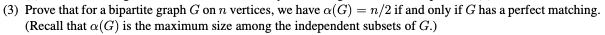Please answer the question and write legibly (3) Prove that for a bipartite graph G on n vertices, we have a(G)- n/2 if and only if G has a perfect matching. (Recall that α(G) is the maximum size among the independent subsets of G.) (3) Prove that for a bipartite graph G on n vertices, we have a(G)- n/2 if and only if G has a perfect matching. (Recall that α(G) is the maximum size among the independent subsets of...

• ### 3. (a) Let Knbe the complete bipartite graph with n vertices in each part of its bipartition, where n 21. Determine the number of perfect matchings of Kn (b) A matching M in a graph Gis ca a mazi...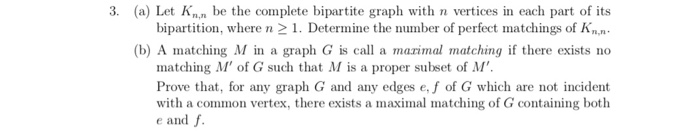3. (a) Let Knbe the complete bipartite graph with n vertices in each part of its bipartition, where n 21. Determine the number of perfect matchings of Kn (b) A matching M in a graph Gis ca a mazimal matching if there exists no matching M' of G such that M is a proper subset of M' Prove that, for any graph G and any edges e,f of G which are not incident with a common vertex, there exists a...

• ### Question 2 (20 marks) (a) How many perfect matchings do each of the following trees Ti and T2 con...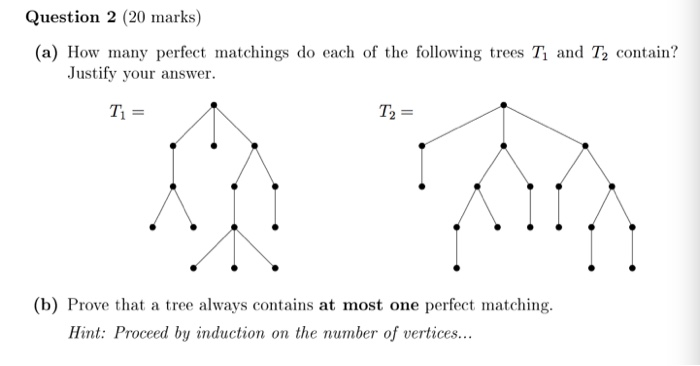Question 2 (20 marks) (a) How many perfect matchings do each of the following trees Ti and T2 contain? Justify your answer. Ti = T2 = (b) Prove that a tree always contains at most one perfect matching Hint: Proceed by induction on the number of vertices... Question 2 (20 marks) (a) How many perfect matchings do each of the following trees Ti and T2 contain? Justify your answer. Ti = T2 = (b) Prove that a tree always contains...

• ### This is a question related to a project I have to do. For this project, I have to choose a Math T...

This is a question related to a project I have to do. For this project, I have to choose a Math Theorem and write about it, discuss its proof, etc. But at the end of the paper I have to include this: "Some part of it must be specific to you, something that you do that is not worked out in any references that you are using. This is very important." My theorem that I chose is the Five Color...

• ### Question 13. Prove that if k is odd and G is a k-regular (k - 1)-edge-connected graph, then G has a perfect matching Question 13. Prove that if k is odd and G is a k-regular (k - 1)-edge-connect...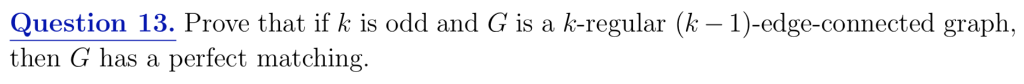Question 13. Prove that if k is odd and G is a k-regular (k - 1)-edge-connected graph, then G has a perfect matching Question 13. Prove that if k is odd and G is a k-regular (k - 1)-edge-connected graph, then G has a perfect matching

• ### Discrete Math: Please help with all parts of question 5. I have included problem 3 to...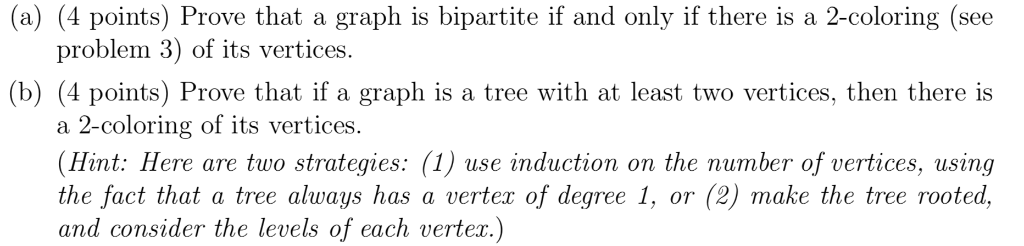Discrete Math: Please help with all parts of question 5. I have included problem 3 to help answer part (a) but I only need help with question 5! 5. 3. (a) (4 points) Prove that a graph is bipartite if and only if there is a 2-coloring (see problem 3) of its vertices. (b) (4 points) Prove that if a graph is a tree with at least two vertices, then there is a 2-coloring of its vertices. (Hint: Here are...

• ### I have to factor this equation, which is a perfect square: 2p^2+4p+1 How do I do this

I have to factor this equation, which is a perfect square:2p^2+4p+1How do I do this?

• ### I have to graph this problem and not sure how to do it

I have to graph this problem and not sure how to do it. Graph y=3x

• ### a graph theory homework questions parts c,d,e,f 6. Let G be the fllowing graph: 1) Fig, 7.7.1 (n) Does G have a perfect matching? (b) Find four maximum matchings in G. (c) Is there any maximum mat...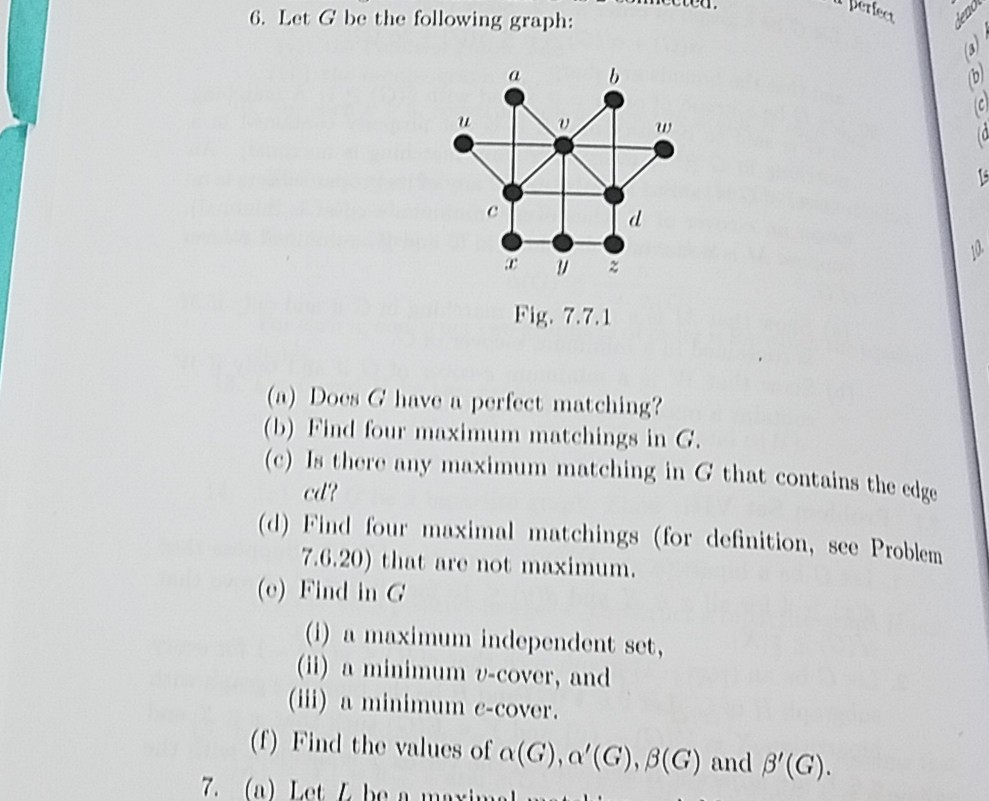a graph theory homework questions parts c,d,e,f 6. Let G be the fllowing graph: 1) Fig, 7.7.1 (n) Does G have a perfect matching? (b) Find four maximum matchings in G. (c) Is there any maximum matching in G that contains the edge cl? (d) Find four maximal matchings (for definition, see Problem 7.6.20) that are not maximum. (e) Find in G (1) a maximum independent set, (ii) a minimum v-cover, and iii) n minimum c-cover. (f) Find the values...

Need Online Homework Help?